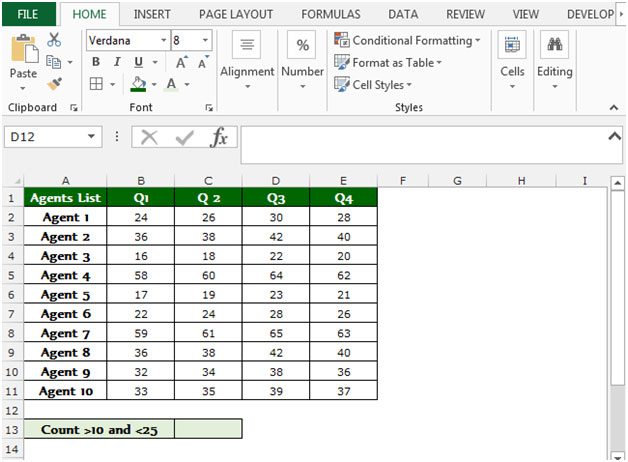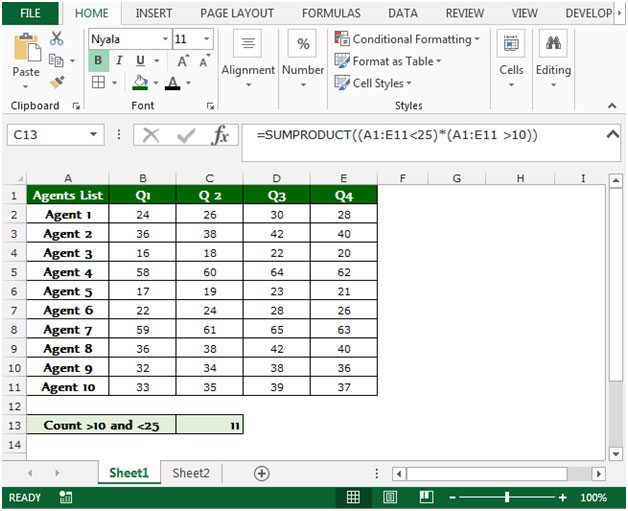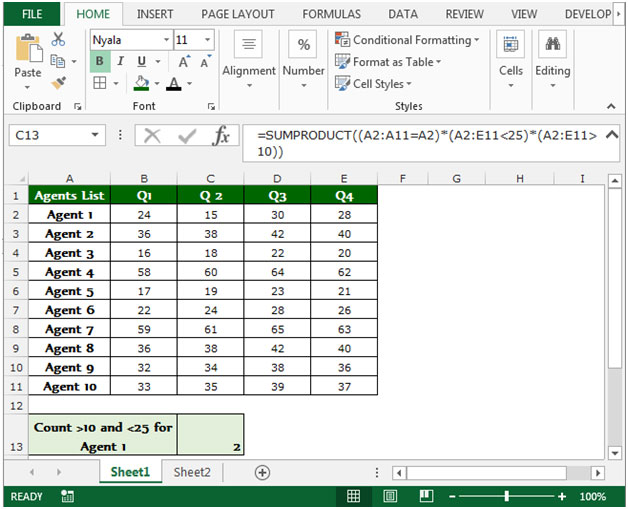# Count the Number of Values between a Lower and Upper Limit in Microsoft Excel

In this article, we will learn how to count the number of values between a lower and upper limit. We will use “SUMPRODUCT” function in Microsoft Excel to get the desired outcome.

SUMPRODUCT: This function is used for adding all the numbers in a range of cells.

The syntax of SUMPRODUCT formula:-  =SUMPRODUCT(array1, [array2],…..)

Let’s take an example to understand how we can count the number of values between a lower and upper limit in Microsoft Excel.

We have table in Range “A1 to E11” in which column A contains Agent list, column B contains no. of sales for Q1, column C contains no. of sales for Q2, column D Q3 and column E contains for Q4.

In this data, we want to count the number of agents who are having the score greater than to 10 and less than 25.To count the number of value between a lower and upper limit in Microsoft Excel, follow below given steps:-

• Select the cell C13.
• Enter the formula in cell =SUMPRODUCT((A1:E11<25)*(A1:E11 >10))
• Press Enter on your keyboard.
• The function will return 11, which means 11 sales number are there between the range of 10-25.What if we require the same for a particular agent?

Follow below given formula to return the no. of sales for agent between the range of 10-25:-

• Select the cell C13.
• =SUMPRODUCT((A2:A11=A2)*(A2:E11<25)*(A2:E11>10))
• Press Enter on your keyboard.
• The function will return 2, which means 2 sales numbers are for Agent 1 in the range of 10-25.Conclusion:- This is all about that how we can count the number of values between upper and lower limit by using the SUMPRODUCT function in Microsoft Excel.